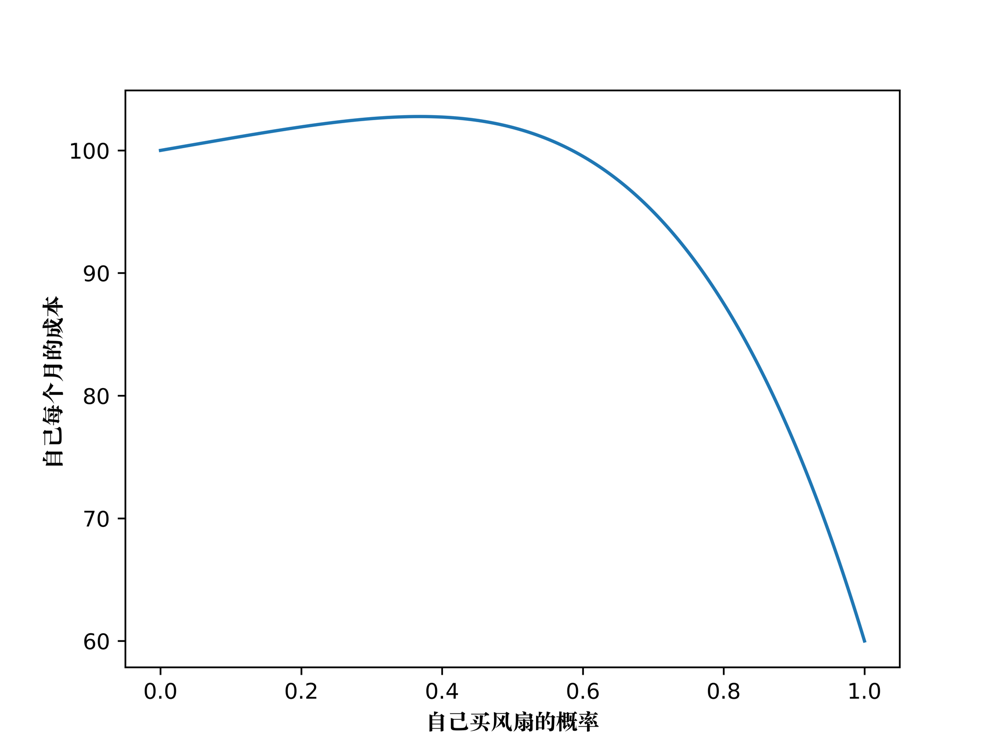# 我觉得这个世界上没有理性的集体······

## 为什么我们学校少有人买风扇

其他三个人
买电风扇 不买电风扇

不买电风扇 100 100

其他三个人
买电风扇（$\alpha^3$） 不买电风扇（$1 - \alpha^3$）

不买电风扇($1-\alpha$) 100 100

\begin{align} f(\alpha) = & \alpha \times \left[ 60 \times \alpha^3 + 110 \times (1-\alpha^3) \right] \\ & + (1- \alpha) \times\left[ 100 \times \alpha^3 + 100 \times (1-\alpha^3) \right] \end{align}

自己的决策目标是：
$$\underbrace{ min{ f(\alpha) } }_{选择\alpha} \\ 其中：\alpha \in [0, 1]$$## 集体一定是非理性的

$$个体信息 = 个体行为 \tag{1}$$

$$\sum{个体行为} = 集体行为 \tag{2}$$

$$\sum{个体信息} < 集体信息 \tag{3}$$

$$集体行为 < 集体信息 \tag{4}$$